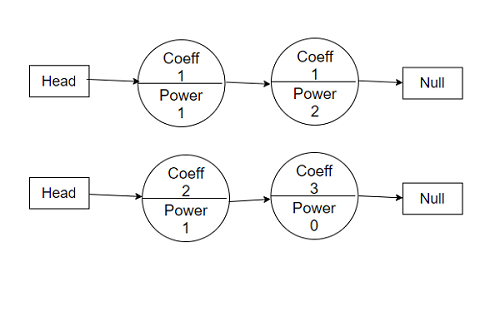# Program to add two polynomials given as linked lists using Python

Suppose, we are given two polynomials and we have to find out the addition of the two polynomials. The polynomials have to be represented as linked lists; the terms of the polynomials will be represented as a linked list node. Each linked list node will contain the coefficient value, power value, and the pointer to the next linked list node. We have to return a third linked list which is the addition of two linked list polynomials.

So, if the input is like1x^1 + 1x^2 = 0 and 2x^1 + 3x^0 = 0,

then the output will be 3x^1 + 1x^2 + 3x^0 = 0

To solve this, we will follow these steps −

• dummy := a new polynomial node

• node := a new polynomial node

• while poly1 and poly2 is not empty, do

• if power of poly1 > power of poly2, then

• next of node := poly1

• node := poly1

• poly1 := next of poly1

• otherwise when power of poly1 < power of poly2, then

• next of node := poly2

• node := poly2

• poly2 := next of poly2

• otherwise,

• coef := coefficient of poly1 + coefficient of poly2

• if coef is non-zero, then

• poly1 := next of poly1

• poly2 := next of poly2

• next of node := poly1 or poly2

• return next of dummy

Let us see the following implementation to get better understanding −

## Example

class polynomial:
def __init__(self, coeff = 0, pow = 0, nxt = None):
self.coefficient = coeff
self.power = pow
self.next = nxt
def create_poly(expression):
for element in expression:
else:
while temp.next != None:
temp = temp.next
if temp.next == None:
temp.next = polynomial(element, element)
while temp.next != None:
print(str(temp.coefficient) + 'x^' + str(temp.power), end = ' + ')
temp = temp.next
if temp.next == None:
print(str(temp.coefficient) + 'x^' + str(temp.power), end=' = 0')
def solve(poly1, poly2):
dummy = node = polynomial()
while poly1 and poly2:
if poly1.power > poly2.power:
node.next = node = poly1
poly1 = poly1.next
elif poly1.power < poly2.power:
node.next = node = poly2
poly2 = poly2.next
else:
coef = poly1.coefficient + poly2.coefficient
if coef: node.next = node = polynomial(coef, poly1.power)
poly1 = poly1.next
poly2 = poly2.next
node.next = poly1 or poly2
return dummy.next
poly1 = create_poly([[1,1], [1,2]])
poly2 = create_poly([[2,1], [3, 0]])
poly3 = solve(poly1, poly2)
show_poly(poly3)

## Input

poly1 = create_poly([[1,1], [1,2]])
poly2 = create_poly([[2,1], [3, 0]])

## Output

3x^1 + 1x^2 + 3x^0 = 0

Updated on: 28-May-2021

2K+ Views